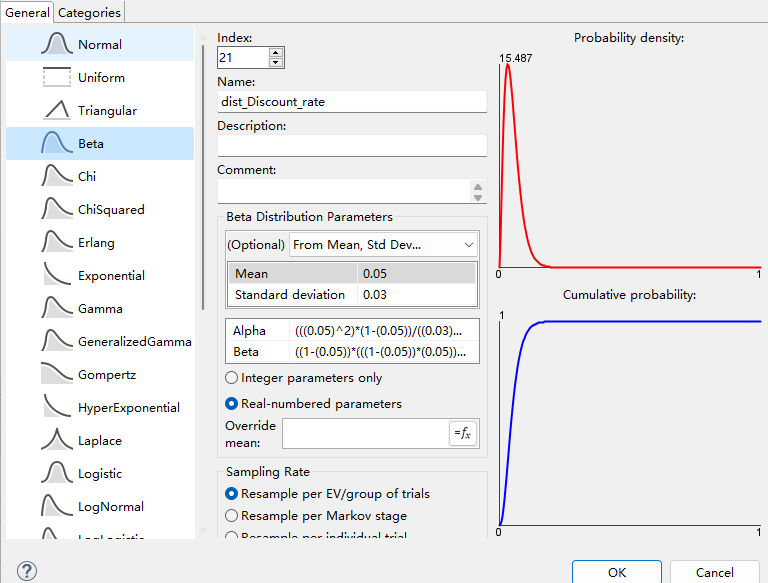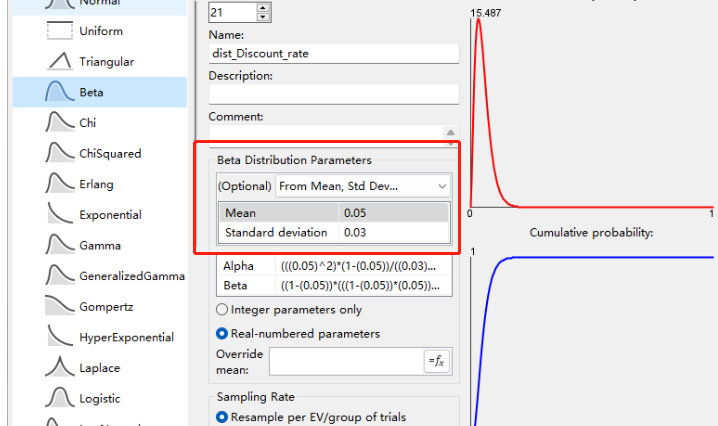# How to set a distribution for discount rate ？

Dear All,

How to set a distribution for discount rate in Treeage Pro? It obeys the beta distribution. The value is 0.05, ranging from 0 to 0.08. I tried setting it up like the one in the picture below, but I think it doesn't work.Sincerely，

Wang Yang

• Wang

I assume you want to set up a discount rate to use in your model. The distribution image you have sent above looks correct if you want the discount rate to be resampled in Probabilistic Sensitivity Analysis. In the distributions view use the GraphIt button to see what the sample will look like for the distribution you have created.

The next issue is how you use the discount rate you have created. My first suggestion is to use Global Discounting as this will appropriately take account of the time cycle you have for your model. You can choose which payoffs to apply the discounting too, and you can enter the discount rate as the distribution you have defined.

Belinda

• Dear Belinda，

Thanks for your reply.  As you said，I want to perform a Probabilistic Sensitivity Analysis. The discount rate is 0.05 and range from 0 to 0.08. What values can I set the mean and standard deviation to represent this distribution.Sincerely，

Wang Yang

• You might want to use a Pert Distribution where you can adjust the value continuously within a range.

Once you have the right distribution, then you will need to enter it into Tree Prefs > Payoffs > Global Discounting for each payoff set you want discounted.